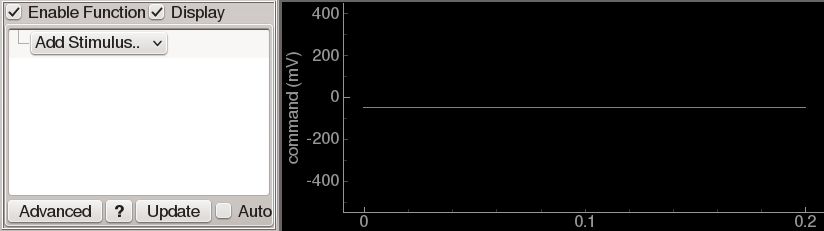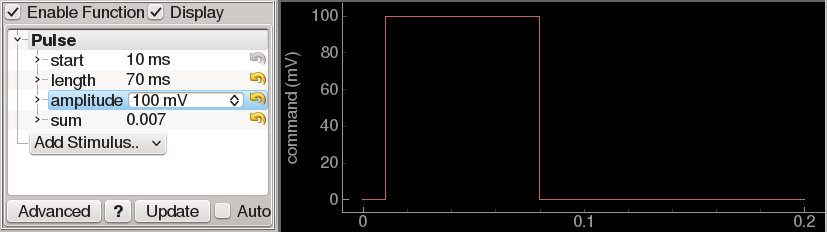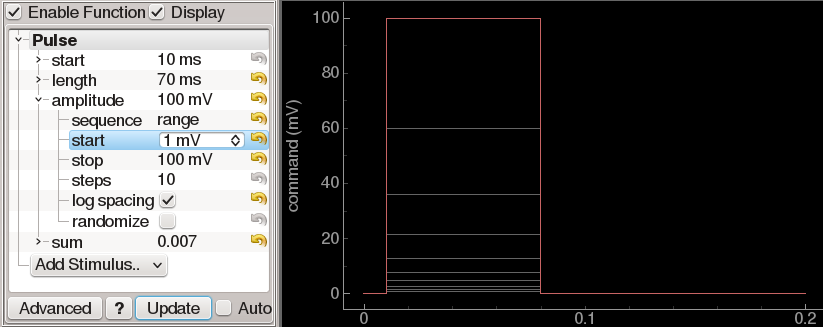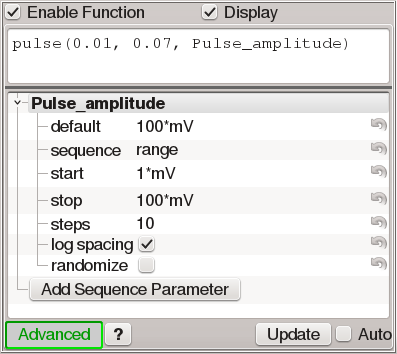# Function generators¶

Many situations in ACQ4 ask the user to define a waveform that will be sent to an analog or digital channel on a DAQ device. For this purpose, ACQ4 uses a general-purpose function generator control accompanied by a plot displaying the output of the generator:Function generators have two modes of operation:

• Simple mode allows the construction of waveforms by selecting one or more elements to add to the waveform and adjusting the parameters for each element. This mode is rather limited in its capabilities (currently, the output may be the sume of one or more square pulses or pulse trains), but is simple to use and generates a metadata structure that can be easily parsed by analysis software.

• Advanced mode allows the user to enter a Python expression that will be evaluated to yield the waveform output. This mode is far more flexible in the types of waveform it can generate, but requires some minimal knowledge of the Python language. In some cases, it also presents a difficult analysis problem because a single waveform may be specified in many different ways. The result is that analysis software may be required to either parse complex Python statements or directly analyze the waveform to determine the stimulation parameters used.

All function generators have the following controls:

• Enable function is used to enable or disable the function generator output. In the context of the Task Runner, a disabled channel is simply ignored when configuring the DAQ to execute a task.

• Display specifies whether the functions generated should be plotted.

• Advanced determines whether the function generator is operating in advanced or simple mode.

• ? can be pressed to display reference documentation related to the function generator.

• ! can be pressed to display error information about the current function being generated.

• Update causes the function generator output to be replotted.

• Auto causes updates to be processed automatically when changes are made to the function.

## Simple mode¶

In simple mode, the generator displays a dropdown menu labeled Add Stimulus. Selecting an item from the list causes a new instance of that item to be added to the total waveform. Multiple items may be added to build up more complex waveforms. Each item is added with a unique name and may be renamed or removed by right-clicking on this name. Currently, only two item types are supported in simple mode: Pulse and Pulse train.Pulse adds a single square pulse to the waveform. It has four parameters that may be set to define the shape of the pulse:

• start determines the time of the beginning of the pulse.

• length determines the duration of the pulse.

• amplitude determines the amplitude of the pulse from baseline.

• sum determines the integral of the pulse amplitude over time.

These four parameters overspecify the shape of the pulse. Thus, changing length or amplitude will also cause the sum to change. By default, changing the sum causes length to change accordingly. However, the sum parameter includes a sub-parameter affect that determines this behavior. Sub-parameters may be accessed by clicking the triangle or + icon adjacent to the parameter name.

Pulse train adds a sequence of square pulses to the waveform. Its parameters are as follows:

• start determines the time of the beginning of the first pulse.

• length determines the duration of each pulse.

• amplitude determines the amplitude of each pulse from baseline.

• sum determines the integral of each pulse amplitude over time.

• period determines the duration from the start of one pulse to the start of the next.

• pulse_number determines the number of pulses in the train.

The length, amplitude, and sum parameters have the same behavior as described above for Pulse.

## Waveform sequences¶

Function generators are also used to design sequences of waveforms with one or more parameters that vary for each point in the sequence. In simple mode, each of the paraneters that define a waveform element may be expanded to reveal a set of sequencing controls that determine whether and how a parameter should be handled in a sequence:Sequences may be specified either as a range or a list. If range is selected, then several parameters are shown which determine how the sequence is constructed:

• start is the first value in the sequence.

• stop is the last value in the sequence.

• steps is the number of values in the sequence.

• log spacing indicates whether the intervals between sequence values are linear or logarithmic.

• randomize indicates whether the order of the sequence should be shuffled.

Alternately, selecting list gives a simple text box in which a comma-separated list of values may be specified.

The waveform is generated once for each value in the sequence and plotted in grey. For example, the figure above shows a pulse that sequences its amplitude logarithmically from 1 mV to 100 mV in 10 steps. The red plot line shows the function evaluated using the default parameters for all values (in this case, 100 mV), ignoring any sequence specifications. If multiple parameters are sequenced, then the function is generated once per combination of sequence values.

In the context of the Task Runner sequencing system, each sequenced parameter creates a new entry in the sequence parameter list. When Test or Record Sequence is clicked there, the task is executed once for each combination of sequence values (grey plot lines). When Test Single or Record Single is clicked, the task is executed only once using the default values (red plot line).

Clicking the Advanced button causes the user interface to display a text box in which a python expression or statements may be written. If a waveform was already specified in simple mode, then clicking Advanced will automatically generate an equivalent function (but note that the translation does not work in the opposite direction; changes made in advanced mode will not carry over when switching back to simple mode).If a Python expression is supplied, it must evaluate to a NumPy array with the correct number of samples. Alternatively, multiple Python statements may be given, ending in a return statement that returns the output array. The values in the array must always be expressed as unscaled units (eg. amperes instead of nano- or picoamperes). This is done to avoid any ambiguity about the required scaling in different contexts. The environment for evaluating the function is defined as follows:

1. Global variables `nPts` and `rate` are defined indicating the required number of points and sample rate.

2. ACQ4’s unit symbols are imported into the global namespace, allowing the code to be written unambiguously with more ‘naturally’ scaled values.

3. NumPy is imported as ‘np’ in the global namespace. This provides a large collection of array and numerical functions.

4. Several convenience functions are defined that simplify the construction of common waveform components:

• **steps**(times, values, [base=0.0])

• **pulse**(times, widths, values, [base=0.0])

• **sineWave**(period, amplitude=1.0, phase=0.0, start=0.0, stop=None, base=0.0)

• **squareWave**(period, amplitude=1.0, phase=0.0, duty=0.5, start=0.0, stop=None, base=0.0)

• **sawWave**(period, amplitude=1.0, phase=0.0, start=0.0, stop=None, base=0.0)

• **listWave**(period, values, phase=0.0, start=0.0, stop=None, base=0.0)

More information about these functions is available by clicking the ? button at the bottom of the function generator.

The Add Sequence Parameter button creates a new global variable which may be used in the function. In the example figure above, the `Pulse_amplitude` variable is automatically sequenced from 1 mV to 100 mV in 10 logarithmically-spaced steps. Specifying the sequence values to use works almost exactly the same as described above for simple mode. The only major difference is that the values entered for each parameter are also evaluated as python expressions.

Square pulse waveform using the built-in `pulse` function:

```pulse(times=10*ms, widths=5*ms, values=-10*mV)
```

The same square pulse waveform, done without the built-in `pulse` function:

```data = np.zeros(nPts)
start = 10*ms * rate
stop = start + 5*ms * rate
data[start:stop] = -10*mV
return data
```

Load waveform from binary data file:

```np.fromfile('stim.dat', dtype=np.float32)
```

## Stored data format¶

Function generators create a standard metadata format that describes all of the parameters it uses when constructing the output waveform. Devices and modules that store data based on a function generator will usually store this metadata structure as well. The structure follows:

• stimuli: A hierarchy of parameters describing each of the simple-mode components that constructed the complete waveform, including the names of the components, their configuration parameters, and any sequencing settings.

• function: The python code that was used to generate the waveform.

• params: The sequence parameters that were used with the Python function.

• advancedMode: (bool) whether the function generator was being used in advanced mode.